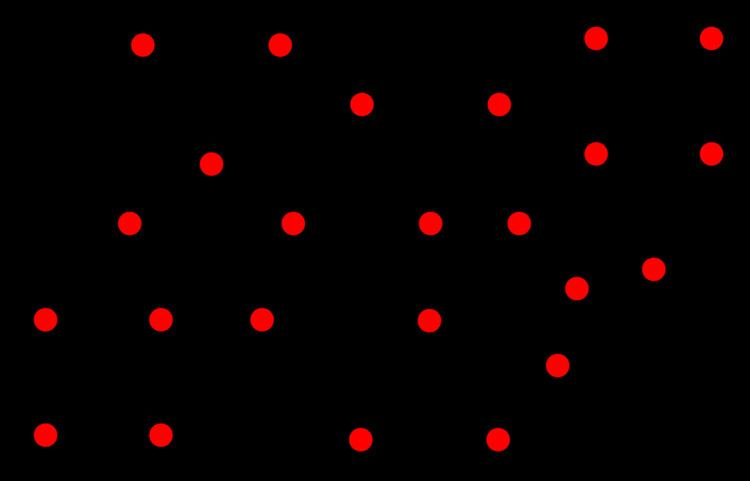# Block graph

Updated on
Covid-19In graph theory, a branch of combinatorial mathematics, a block graph or clique tree is a type of undirected graph in which every biconnected component (block) is a clique.

## Contents

Block graphs are sometimes erroneously called Husimi trees (after Kôdi Husimi), but that name more properly refers to cactus graphs, graphs in which every nontrivial biconnected component is a cycle.

Block graphs may be characterized as the intersection graphs of the blocks of arbitrary undirected graphs.

## Characterization

Block graphs are exactly the graphs for which, for every four vertices u, v, x, and y, the largest two of the three distances d(u,v) + d(x,y), d(u,x) + d(v,y), and d(u,y) + d(v,x) are always equal.

They also have a forbidden graph characterization as the graphs that do not have the diamond graph or a cycle of four or more vertices as an induced subgraph; that is, they are the diamond-free chordal graphs. They are also the Ptolemaic graphs (chordal distance-hereditary graphs) in which every two nodes at distance two from each other are connected by a unique shortest path, and the chordal graphs in which every two maximal cliques have at most one vertex in common.

A graph G is a block graph if and only if the intersection of every two connected subsets of vertices of G is empty or connected. Therefore, the connected subsets of vertices in a connected block graph form a convex geometry, a property that is not true of any graphs that are not block graphs. Because of this property, in a connected block graph, every set of vertices has a unique minimal connected superset, its closure in the convex geometry. The connected block graphs are exactly the graphs in which there is a unique induced path connecting every pair of vertices.

## Related graph classes

Block graphs are chordal and distance-hereditary. The distance-hereditary graphs are the graphs in which every two induced paths between the same two vertices have the same length, a weakening of the characterization of block graphs as having at most one induced path between every two vertices. Because both the chordal graphs and the distance-hereditary graphs are subclasses of the perfect graphs, block graphs are perfect.

Every tree, cluster graph, or windmill graph is a block graph.

Every block graph has boxicity at most two.

Block graphs are examples of pseudo-median graphs: for every three vertices, either there exists a unique vertex that belongs to shortest paths between all three vertices, or there exists a unique triangle whose edges lie on these three shortest paths.

The line graphs of trees are exactly the block graphs in which every cut vertex is incident to at most two blocks, or equivalently the claw-free block graphs. Line graphs of trees have been used to find graphs with a given number of edges and vertices in which the largest induced subgraph that is a tree is as small as possible.

## Block graphs of undirected graphs

If G is any undirected graph, the block graph of G, denoted B(G), is the intersection graph of the blocks of G: B(G) has a vertex for every biconnected component of G, and two vertices of B(G) are adjacent if the corresponding two blocks meet at an articulation vertex. If K1 denotes the graph with one vertex, then B(K1) is defined to be the empty graph. B(G) is necessarily a block graph: it has one biconnected component for each articulation vertex of G, and each biconnected component formed in this way must be a clique. Conversely, every block graph is the graph B(G) for some graph G. If G is a tree, then B(G) coincides with the line graph of G.

The graph B(B(G)) has one vertex for each articulation vertex of G; two vertices are adjacent in B(B(G)) if they belong to the same block in G.

## References

Similar Topics
Florentino García Martínez
Carmela Corren
Victor DelCampo
Topics